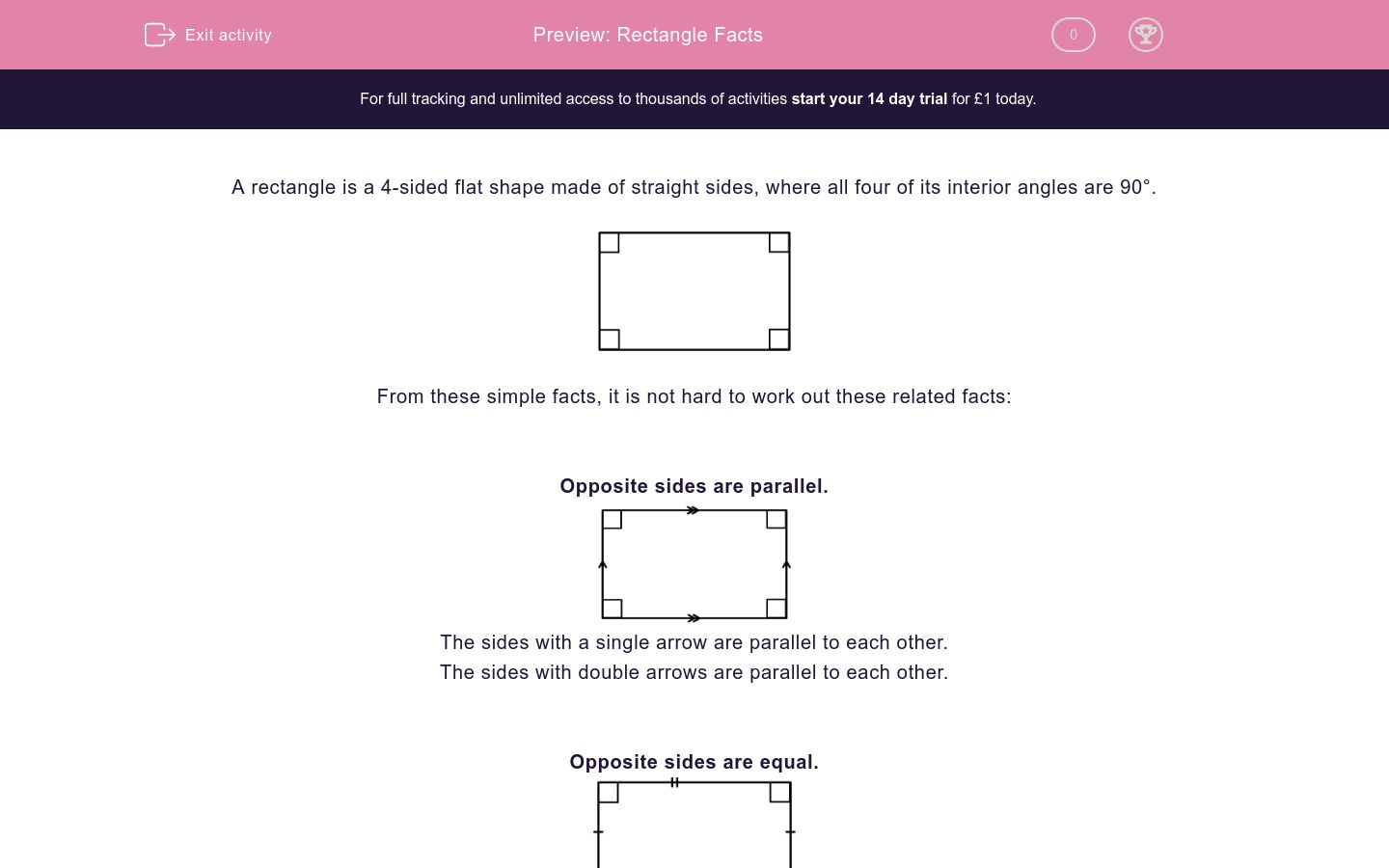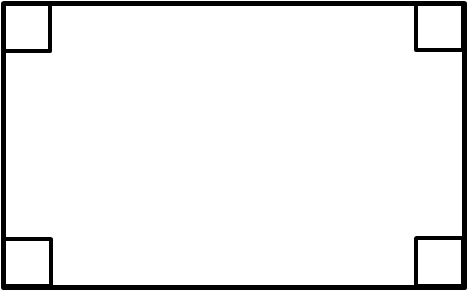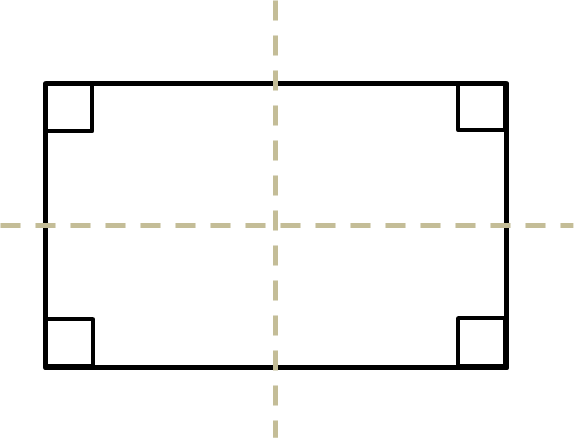# Rectangle Facts

In this worksheet, students must use the properties of rectangles to deduce related facts.Key stage:  KS 2

Curriculum topic:   Maths and Numerical Reasoning

Curriculum subtopic:   Symmetry

Difficulty level:### QUESTION 1 of 10

A rectangle is a 4-sided flat shape made of straight sides, where all four of its interior angles are 90°.From these simple facts, it is not hard to work out these related facts:

Opposite sides are parallel.The sides with a single arrow are parallel to each other.

The sides with double arrows are parallel to each other.

Opposite sides are equal.The sides with a single dash are equal in length to each other.

The sides with a double dash are equal in length to each other.

There are 2 lines of symmetry.True or false?

A rectangle has 4 right angles.

true

false

True or false?

A rectangle has 2 diagonals.true

false

True or false?

A rectangle has 4 lines of symmetry.true

false

What is the total of the interior angles of a rectangle in degrees?

(Just write the number.)

True or false?

A rectangle can have 6 sides.

true

false

True or false?

All squares are rectangles.

true

false

True or false?

All rectangles are squares.

true

false

True or false?

Opposite sides of a rectangle are equal in length.

true

false

True or false?

Opposite sides of a rectangle never meet even if they are made longer.

true

false

True or false?

The perimeter of this rectangle is 25 cm.true

false

• Question 1

True or false?

A rectangle has 4 right angles.

true
EDDIE SAYS
A rectangle has 4 interior angles, each of 90°.
• Question 2

True or false?

A rectangle has 2 diagonals.true
EDDIE SAYS
Diagonals go from one corner to its opposite corner.
• Question 3

True or false?

A rectangle has 4 lines of symmetry.false
EDDIE SAYS
Try folding a piece of paper along these lines.
The diagonals are not lines of symmetry.
• Question 4

What is the total of the interior angles of a rectangle in degrees?

(Just write the number.)

360
EDDIE SAYS
4 × 90° = 360°
• Question 5

True or false?

A rectangle can have 6 sides.

false
EDDIE SAYS
Rectangles have 4 sides.
• Question 6

True or false?

All squares are rectangles.

true
EDDIE SAYS
A square is a 4-sided flat shape made of straight sides, where all four of its interior angles are 90°. So it is a rectangle.
• Question 7

True or false?

All rectangles are squares.

false
EDDIE SAYS
Not all rectangles have 4 sides of equal length.
• Question 8

True or false?

Opposite sides of a rectangle are equal in length.

true
EDDIE SAYS
Look at the diagram in the introduction.
• Question 9

True or false?

Opposite sides of a rectangle never meet even if they are made longer.

true
EDDIE SAYS
Opposite sides are parallel and so never meet.
• Question 10

True or false?

The perimeter of this rectangle is 25 cm.false
EDDIE SAYS
Opposite sides are equal in length, so there are two sides of length 10 cm and two sides of length 15 cm.
The perimeter is 10 + 15 + 10 + 15 = 50 cm.
---- OR ----

Sign up for a £1 trial so you can track and measure your child's progress on this activity.

### What is EdPlace?

We're your National Curriculum aligned online education content provider helping each child succeed in English, maths and science from year 1 to GCSE. With an EdPlace account you’ll be able to track and measure progress, helping each child achieve their best. We build confidence and attainment by personalising each child’s learning at a level that suits them.

Get started